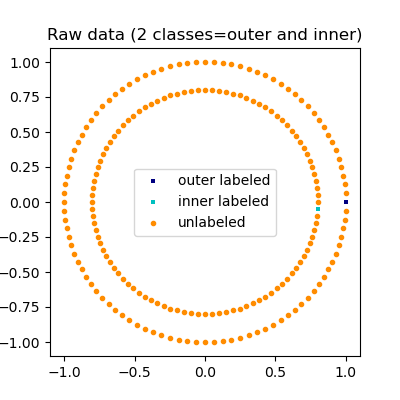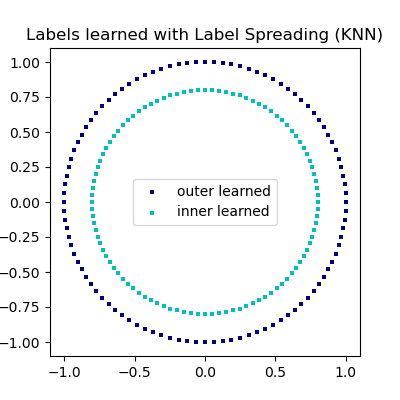# Label Propagation learning a complex structure¶

Example of LabelPropagation learning a complex internal structure to demonstrate “manifold learning”. The outer circle should be labeled “red” and the inner circle “blue”. Because both label groups lie inside their own distinct shape, we can see that the labels propagate correctly around the circle.

# Authors: Clay Woolam <clay@woolam.org>
#          Andreas Mueller <amueller@ais.uni-bonn.de>


We generate a dataset with two concentric circles. In addition, a label is associated with each sample of the dataset that is: 0 (belonging to the outer circle), 1 (belonging to the inner circle), and -1 (unknown). Here, all labels but two are tagged as unknown.

import numpy as np
from sklearn.datasets import make_circles

n_samples = 200
X, y = make_circles(n_samples=n_samples, shuffle=False)
outer, inner = 0, 1
labels = np.full(n_samples, -1.0)
labels = outer
labels[-1] = inner


Plot raw data

import matplotlib.pyplot as plt

plt.figure(figsize=(4, 4))
plt.scatter(
X[labels == outer, 0],
X[labels == outer, 1],
color="navy",
marker="s",
lw=0,
label="outer labeled",
s=10,
)
plt.scatter(
X[labels == inner, 0],
X[labels == inner, 1],
color="c",
marker="s",
lw=0,
label="inner labeled",
s=10,
)
plt.scatter(
X[labels == -1, 0],
X[labels == -1, 1],
color="darkorange",
marker=".",
label="unlabeled",
)
_ = plt.title("Raw data (2 classes=outer and inner)")The aim of LabelSpreading is to associate a label to sample where the label is initially unknown.

from sklearn.semi_supervised import LabelSpreading


LabelSpreading(alpha=0.8, kernel='knn')
In a Jupyter environment, please rerun this cell to show the HTML representation or trust the notebook.

Now, we can check which labels have been associated with each sample when the label was unknown.

output_labels = label_spread.transduction_
output_label_array = np.asarray(output_labels)
outer_numbers = np.where(output_label_array == outer)
inner_numbers = np.where(output_label_array == inner)

plt.figure(figsize=(4, 4))
plt.scatter(
X[outer_numbers, 0],
X[outer_numbers, 1],
color="navy",
marker="s",
lw=0,
s=10,
label="outer learned",
)
plt.scatter(
X[inner_numbers, 0],
X[inner_numbers, 1],
color="c",
marker="s",
lw=0,
s=10,
label="inner learned",
)